# Damage Stats - An easier way to calculate damage

#### X-Act

##### np: Biffy Clyro - Shock Shock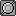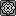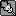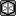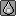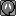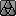Here's an easy way to get an excellent approximation of the damage dealt by a Pokemon to another. The method given below doesn't even require that you know the actual stats of the Pokemon in question - only their base stats.

First of all, a chart converting a Base stat to the equivalent Damage stat:

Code:
``````   BStat   DStat
-----------------
5    2.19
10    2.67
15    3.14
20    3.62
25    4.10
30    4.57
35    5.05
40    5.52
45    6.00
50    6.48
55    6.95
60    7.43
65    7.90
70    8.38
75    8.86
80    9.33
85    9.81
90   10.29
95   10.76
100   11.24
105   11.71
110   12.19
115   12.67
120   13.14
125   13.62
130   14.10
135   14.57
140   15.05
145   15.52
150   16.00
160   16.95
165   17.43
170   17.90
180   18.86
190   19.81
200   20.76
230   23.62
250   25.52
255   26.00
Stat ±2   ±0.19
Stat ±4   ±0.38
252 EVs   +3.00
HP   +5.00
Atk/SpA   x4.00``````
The above table is to convert the Def or SpD base stat. To convert the base HP stat, just add 5 to the corresponding number, and if you want to convert the base Atk or SpA stat, just multiply the number by 4. You'll first need to do the additions and subtractions before performing any multiplications or divisions in order for the method to work, however.

If the stat you want is not on the list (say you have a Pokemon with 88 SpA or 144 HP or 77 SpD or whatever), you add or subtract 0.19 if you want to add or subtract 2 from the stat, and twice that (0.38) if you want to add or subtract 4.

So 77 SpD corresponds to 8.86, the stat for 75, + 0.19 = 9.05.
144 HP corresponds to 15.05, the stat for 140, + 0.38, + 5 (since this is HP) = 20.43.
88 SpA corresponds to 10.29, the stat for 90, -0.19, x4 (since this is SpA) = 40.4 (remember you first perform the subtraction and then the multiplication).

If you have 252 EVs in a stat, you add 3. Simple as that. This means that you add 1/21, or 0.0476, for every 4 EVs you have. You can also understand it as dividing the EVs by 84 and adding that to the damage stat.

Any boosts in damage or in stats, be them due to nature, STAB, item, ability, or whatever are multiplied normally.

After doing all this, the percentage maximum damage dealt is then simply

Code:
``Max Damage = (D[S]Atk x MovePower) / (DHP x D[S]Def)``
The min damage is just 0.85 multiplied by the max damage. You don't even need to convert it to a percentage; the answer is already a percentage!

Let's give an example.

Quoting part of a post by MoP:
438 attack vs 276 defense, 120 power(*1.5), 321 max HP: 63.86% - 75.08%

438 for Modest Special Attack. Palkia's min Special Defense is 276 along with 321 HP. The *1.5 represents Choice Specs.
So we have Choice Specs Modest Kyogre with 252 SpA EVs using Thunder against 0/0 Palkia. Notice that he had to find Kyogre's attacking stat (438) and Palkia's HP and SpD stats (321 and 276).

The method above would work as follows:

Kyogre's base SpA is 150. 150 corresponds to a damage stat of 16.00. We add 3 due to having 252 EVs, so we have 19. We multiply by 4 since this is the SpA stat, so we have 76. We multiply by 1.1 since this is Modest, so we have 83.6. We then multiply by 1.5 due to Choice Specs, so we have 125.4.

Palkia's base HP is 90. 90 corresponds to a damage stat of 10.29. We add 5 since this is the HP stat, so we have 15.29.

Palkia's base SpD is 120. 120 corresponds to a damage stat of 13.14.

So max damage is (125.4 x 120) / (15.29 x 13.14) = 74.90%. Min damage is 74.9% x 0.85 = 63.66%. So I'm about 0.2% off the real damage.

Another example (taken from a post by Gothic Togekiss:)

CAP6 Thunder Punch vs 124 HP / 252 Def Bold Milotic: 37.57% - 44.20%
We have the latest CAP Pokemon having 110 base Atk with 252 EVs in Atk and a beneficiary nature using Thunderpunch against a 124/252 Bold Milotic. Milotic's base HP and Def are 95 and 79 respectively.

So we have:

CAP6's base Atk is 110, which corresponds to 12.19. We have 252 EVs so we add 3: 15.19. Since this is Atk, we multiply by 4: 60.76. We multiply by 1.1 since it has a beneficial nature: 66.84. We further multiply by 2 since Thunderpunch is supereffective: 133.68.

Milotic's base HP is 95, which corresponds to 10.76. We add 5 since this is HP: 15.76. We also add 124 / 84 = 1.48 since it has 124 HP EVs, getting 17.24.

Milotic's base Def is 79, which corresponds to 8.86 + 0.38 = 9.24. We add 3 since we have 252 EVs: 12.24. We multiply by 1.1 since this is Bold: 13.46.

Hence, the maximum damage would be (133.68 x 75) / (17.24 x 13.46) = 43.21%. The minimum damage would be 43.2 x 0.85 = 36.73%. So we're only 1% off the real damage.

If you want a decent but simple damage calculator using just the base stats of the Pokemon in question, give this a try.

#### Abacus

This looks absurdly useful for on the fly calculations. Cool beans.

#### Malfunction

X-Act you're awesome. Thanks for that.

#### Peachfuzz

Awesome how you can figure things out like this. That's amazing.

This seems very useful. Surprised how accurate it is.

#### SHUCKLE MAN

Wow! That's so cool! Thanks very much! It's good how you think of these things.

#### 1234gjy

So confusing, can someone help me?

BTW, are you really good at math and stuff? How else are you suppose to create all these formulas?

I am confused by how it worked and why it works.

Can you tell me how you made these formulas X-act?

#### Matthew

##### I love weather; Sun for days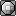BTW, are you really good at math and stuff? How else are you suppose to create all these formulas?
Asking X-Act if he's good at math is like asking an artist if he likes paintings.

#### Articanus

....or asking Michael Jackson if he likes little boys....

anyway, thanks a lot X-Act, you never cease to amaze me! =D

#### TODICHUpwns

great work X-act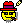#### Wes

Can you tell me how you made these formulas X-act?
Are you really sure you'd want to know? X-Act just knows this stuff... if you don't get the formula itself, I don't think you'll understand the explanation . That's like asking...Gen. some help please
Anyway, great formula X-Act, like always.\$
EDIT: I think he simplified this http://www.smogon.com/dp/articles/damage_formula

#### Matthew

##### I love weather; Sun for daysThat's like asking Colin why he made shoddy... but X-Act will tell you in a nicer way

#### Darth Meanie

Once again, great work X-Act. Took me two readings but I get it. Does it always underestimate damage or does it fluctuate, because both examples have it lower than the actual damage range.

Also, is there a way to quickly find the deviation from the actual value, or would that go back to the actual damage formula.

I'm pretty sure I've misused a few terms, it's been a year since I've taken Calculus.

#### Chinese Dood

Hey! I think this is great! At first I thought: "This doesn't seem that much faster, have to understand and go through quite a bit of calculations still." But, after reading this a second time around, I think I can make some use of this. Thanks!

Also, from what I figured, I think you can just simplify that entire BStat vs DStat table into something like this:

DStat = (36 + 2 * BStat) / 21

I'm not sure if the slight differences in rounding matter though.

#### AhrenElessedil

Wonderful. Simply wonderful.

#### pyrzi

Who X-Act, where do you study this stuff? Awesome!

#### gorm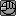from what i can tell you used a logarithmic scale to get base stats in a useable form.. this is exceptionally useful since it's alot more convenient to memorize base stats than to memorize actual stats. phenomenal work.

Everyone here saying awesome, yet I doubt any of them well ever use it.
if by "well" [sic] you mean "us low quality users" then yeah maybe. don't make stupid posts like this.

#### X-Act

##### np: Biffy Clyro - Shock ShockI didn't think that this would be useful, honestly. I just posted it here more as a 'curiosity' than anything else. Glad people find it useful.

Let me answer a few questions:

"Does it always underestimate damage?" I'd say that the answer is probably yes. In fact, if you add 9.52 / DHP to the max damage, you'll get an even better approximation. This means, incidentally, that the lower the base HP, the less accurate the calculation is, so you might want to add that if you're attacking things like Dusknoir and Shuckle.

"Are you really good at math and stuff?" Once again, I'd say that the answer is probably yes.

"Can you tell me how you made these formulas?" Well, sure. But I'll dedicate an entire post for that.

"From what I figured, I think you can just simplify that entire BStat vs DStat table into something like this: DStat = (36 + 2 * BStat) / 21." Very good! That is the exact formula I used to convert them. The reason why this formula is so useful for on-the-fly calculations will be explained later though.

#### Flying Machine

I really find this helpful, for example, I just know CBTar OHKOs Blissey with pursuit, doing <b>185.62-218.38%</b> counting 252 EVs, Adamant and STAB. I think this will be especially useful while calculating, like, 0/0 Mence, taking damage from CB Scizor Punch, with/out Intimidate. Thanks a lot X-Act! :D (although you may not know me because I'm a noob ._. )

BTW it'd be nice if someone makes an XLS with all options, I have one with Choice,Choice+Nature,0 EVs Neutral Nature, 252 EVs + Nature, and plain 0 / 0 for attack, and just HP and Sp/Def Calculator, and I'd like to include EVs into it.

#### Captain Falcon

Cheers, I tried using it earlier on shoddy and I was suprised by how accurate it was. You're a Diamond X-Act!

#### david stone

##### Fast-moving, smart, sexy and alarming.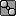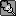Reminds me of these:

#### Darth Meanie

"Does it always underestimate damage?" I'd say that the answer is probably yes. In fact, if you add 9.52 / DHP to the max damage, you'll get an even better approximation. This means, incidentally, that the lower the base HP, the less accurate the calculation is, so you might want to add that if you're attacking things like Dusknoir and Shuckle.

Cool!

... What is DHP?

#### X-Act

##### np: Biffy Clyro - Shock ShockReminds me of these:

It's more similar to the Skarmcents/Blisscents thing than the tiers, since this is not a logarithmic system. However, my system handles EVs much better and it needs only one chart, so it's simpler and can do more.

Cool!

... What is DHP?
DHP is the damage HP stat, which is the number taken from the table plus 5 (plus 3 if you have 252 EVs in HP).

#### Luxormaniac

So DStat = (36 + 2 * BStat) / 21? Thats nice to know- i'd rather play on shoddy with my calculator than have to refer to this thread.

Finally. I always just guessed about OHKO's vs. 2HKO's. This should improve my rating by OVER 9000!!! points!

#### X-Act

##### np: Biffy Clyro - Shock ShockYou can also make the calculation (36 + 2 * BStat) / 21 more rapid by taking the base stat, adding 18, and dividing by 10.5. :)

#### Flying Machine

This is so cool I finally made a one which includes EVs... btw, could someone tell me how to calculate 1-2HKOs? (% chance)
like, whats % of OHKO when taking 84-102% damage from 1 atk.
ty again xact :D